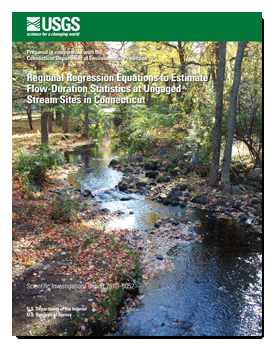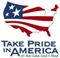Scientific Investigations Report 2010–5052

## Regional Regression Equations to Estimate Flow-Duration Statistics at Ungaged Stream Sites in Connecticut

### ABSTRACTMultiple linear regression equations for determining flow-duration statistics were developed to estimate select flow exceedances ranging from 25- to 99-percent for six “bioperiods”—Salmonid Spawning (November), Overwinter (December–February), Habitat Forming (March–April), Clupeid Spawning (May), Resident Spawning (June), and Rearing and Growth (July–October)—in Connecticut. Regression equations also were developed to estimate the 25- and 99-percent flow exceedances without reference to a bioperiod. In total, 32 equations were developed. The predictive equations were based on regression analyses relating flow statistics from streamgages to GIS-determined basin and climatic characteristics for the drainage areas of those streamgages. Thirty-nine streamgages (and an additional 6 short-term streamgages and 28 partial-record sites for the non-bioperiod 99-percent exceedance) in Connecticut and adjacent areas of neighboring States were used in the regression analysis. Weighted least squares regression analysis was used to determine the predictive equations; weights were assigned based on record length. The basin characteristics—drainage area, percentage of area with coarse-grained stratified deposits, percentage of area with wetlands, mean monthly precipitation (November), mean seasonal precipitation (December, January, and February), and mean basin elevation—are used as explanatory variables in the equations.

Standard errors of estimate of the 32 equations ranged from 10.7 to 156 percent with medians of 19.2 and 55.4 percent to predict the 25- and 99-percent exceedances, respectively. Regression equations to estimate high and median flows (25- to 75-percent exceedances) are better predictors (smaller variability of the residual values around the regression line) than the equations to estimate low flows (less than 75-percent exceedance). The Habitat Forming (March–April) bioperiod had the smallest standard errors of estimate, ranging from 10.7 to 20.9 percent. In contrast, the Rearing and Growth (July–October) bioperiod had the largest standard errors, ranging from 30.9 to 156 percent. The adjusted coefficient of determination of the equations ranged from 77.5 to 99.4 percent with medians of 98.5 and 90.6 percent to predict the 25- and 99-percent exceedances, respectively.

Descriptive information on the streamgages used in the regression, measured basin and climatic characteristics, and estimated flow-duration statistics are provided in this report. Flow-duration statistics and the 32 regression equations for estimating flow-duration statistics in Connecticut are stored on the U.S. Geological Survey World Wide Web application “StreamStats” (http://water.usgs.gov/osw/streamstats/index.html). The regression equations developed in this report can be used to produce unbiased estimates of select flow exceedances statewide.

First posted April 21, 2010

For additional information contact:
Director
Connecticut Water Science Center
U.S. Geological Survey
101 Pitkin Street, East Hartford, CT 06108

(860)291-6740

http://ct.water.usgs.gov

Part or all of this report is presented in Portable Document Format (PDF); the latest version of Adobe Reader or similar software is required to view it. Download the latest version of Adobe Reader, free of charge.

### Suggested citation:

Ahearn, E.A., 2010, Regional regression equations to estimate flow-duration statistics at ungaged stream sites in Connecticut: U.S. Geological Survey Scientific Investigations Report 2010–5052, 45 p., at http://pubs.usgs.gov/sir/2010/5052/.

### Contents

Abstract

Introduction

Purpose and Scope

Physical Setting

Previous Studies

Data-Set Development

Calculation of Flow-Duration Statistics at Streamgages

Drainage-Basin Characteristics of the Streamgages

Regional Regression Analysis

Development of Regression Equations

Assessment of Regression Equations

Final Regression Equations

Limitation of the Regression Equations

Summary

Acknowledgments

References Cited

Appendix 1. Basin characteristics considered for use in regression analysis

Appendix 2. Predictor and explanatory variable names used in StreamStatsU.S. Department of the Interior | U.S. Geological Survey
[an error occurred while processing this directive] URL: http://pubs.usgs.gov